## Wednesday, April 13, 2016

### Estimating Quality of Noise Removal with Dynamic Time Warping: Programmatic Note 18 on Ripples in Mathematics

Problem

This is my programmatic note 18 on Ch. 04, p. 31-34, in "Ripples in Mathematics" by A. Jensen & A. la Cour-Harbo. These notes document my programmatic journey, sometimes easy, sometimes painful, but always joyful, through this great text. My notes are for those who want to use Java as a programming investigation tool of various wavelet algorithms and Octave for plotting the results. You can find my previous notes by searching my blog on "ripples in mathematics." If you are on the same journey, let me know of any bugs in my code or improvements you have found that let us all, fellow travelers on the wavelet road, get better and more beautiful results.

As I was working on Ex. 4.4, p. 34, I started thinking about how one can measure the quality of noise removal in a signal. In particular, suppose there is a signal with some added noise to which the multi-resolution analysis is applied for a specific number of scales. How close is the signal reconstructed from the scalar coefficients (S8, S7, S6, S6, etc) to the signal without any noise.

One method I thought of using is to measure the difference between the original (w/o any noise) and reconstructed signals with dynamic time warping. The exact steps of the method are as follows:  1) do the multi-resolution analysis with forward DWT (CDF or HWT) for a given number of scales on the noisy signal; 2) reconstruct the signal from the appropriate scalar coefficients with inverse DWT; 3) measure the difference between the original and reconstructed signal with dynamic time warping.

Dynamic Time Warping

A repo with my Java implementation of DTW is here. The class TwoDCurveComparator has two methods for comparing two curves with DWT.

public static double compareTwoDCurve(double[] curve_one, double[] curve_two, IDTWSimilarity isim, IWarpingWindow iww) {
return DTW.dpDTW2ColDoubleCost(curve_one, curve_two, isim, iww);

}

public static double compareTwoDCurve(String curveFilePathOne, String curveFilePathTwo, IDTWSimilarity isim, IWarpingWindow iww) {
double[] curve_one = TwoDCurveComparator.fileToPrimitiveDoubleArray(curveFilePathOne);
double[] curve_two = TwoDCurveComparator.fileToPrimitiveDoubleArray(curveFilePathTwo);
final double sim = DTW.dpDTW2ColDoubleCost(curve_two, curve_two, isim, iww);
curve_one = null;
curve_two = null;
return sim;

}

Here is how one can compare two curves using squared euclidian similarity (SquaredEquclideanSim.java), where the first two arguments are the arrays of doubles with self-explanatory names.

double cdf44_dtw_se = DTW.dpDTW2ColDoubleCost(ex_4_4_512_no_noise_p34, ex_4_4_512_with_noise_cdf44_s5_p34,
new SquaredEuclideanSim(), DTW.MaxWW);

double hwt_dtw_se = DTW.dpDTW2ColDoubleCost(ex_4_4_512_no_noise_p34, ex_4_4_512_with_noise_hwt_s5_p34,
new SquaredEuclideanSim(), DTW.MaxWW);

Some Graphs

Below are some signal graphs. Figures 1 and 2 give the original and noisy signals, respectively. Figures 3 - 6 show various reconstructions.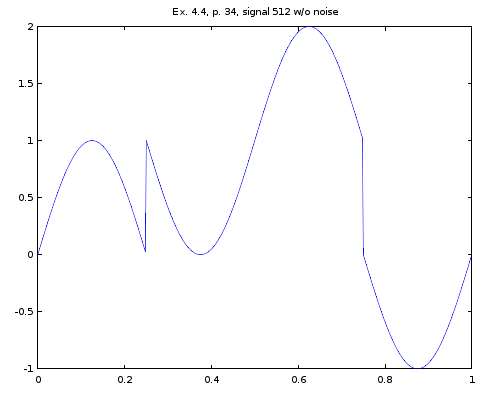Figure 1. Signal from ex. 4.4., p. 34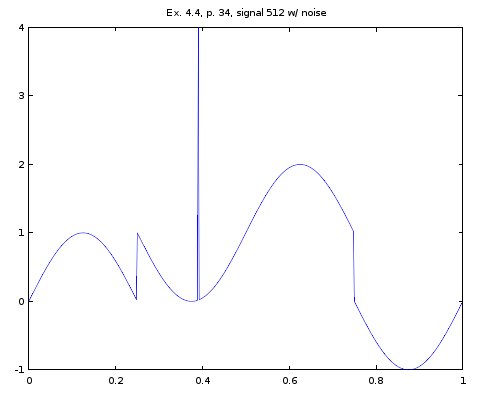Figure 2. Signal from ex. 4.4, p. 34, with a noise spike added at 0.4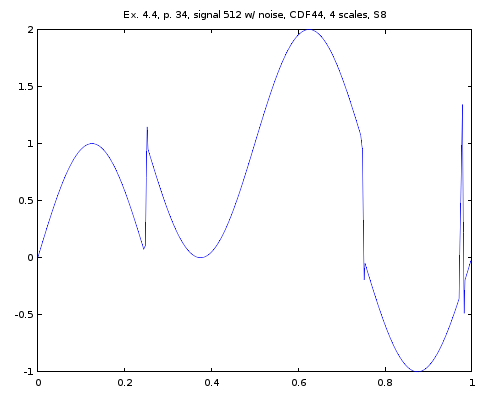Figure 3. CDF44 reconstruction from S8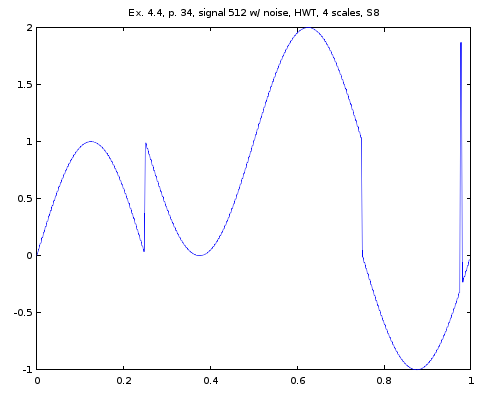Figure 4. HWT reconstruction from S8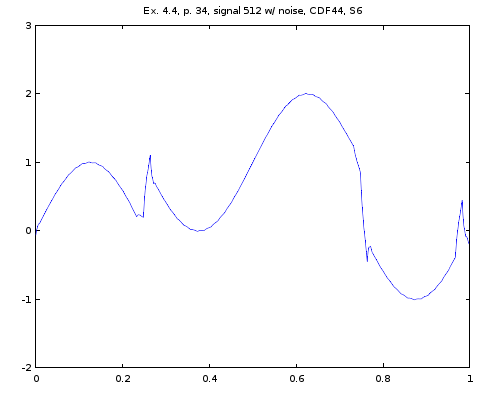Figure 5. CDF44 reconstruction from S6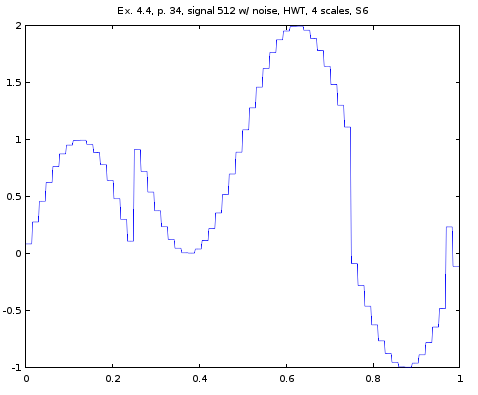Figure 6. HWT signal reconstruction from S6.

Tests

The class TwoDCurveComparator.java has the following methods that measure the quality of noise removal with difference numbers of scales from the signal in Ex. 4.4 on p. 34: test_ex_4_4_p34_s8(), test_ex_4_4_p34_s7(), test_ex_4_4_p34_s6(), and test_ex_4_4_p34_s5(). These methods display the values of various similarity metrics between the original and reconstructed signals. Below are some DWT values between the original and reconstructed signals. In the notation below, CDF(4, 4) or HWT denote the DWT, S8, S7, and S6 denote the scalar values at a specific scale, the number to the right side of the equality sign is the value of the DTW between the reconstructed signal and the signal in Fig. 2.

CDF(4, 4), S8  == 3.9685421518395367
HWT, S8   == 7.684719899677848
CDF(4, 4), S7      == 1.688302564009318
HWT, S7 == 3.4639739360892845
CDF(4, 4), S6  == 1.7377716142440158
HWT, S6  == 2.0367401581624174